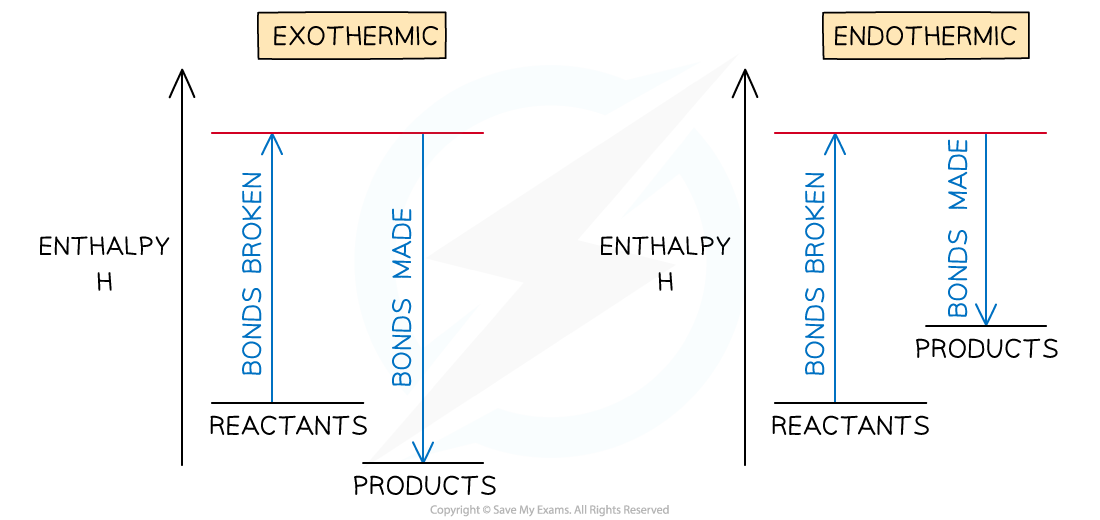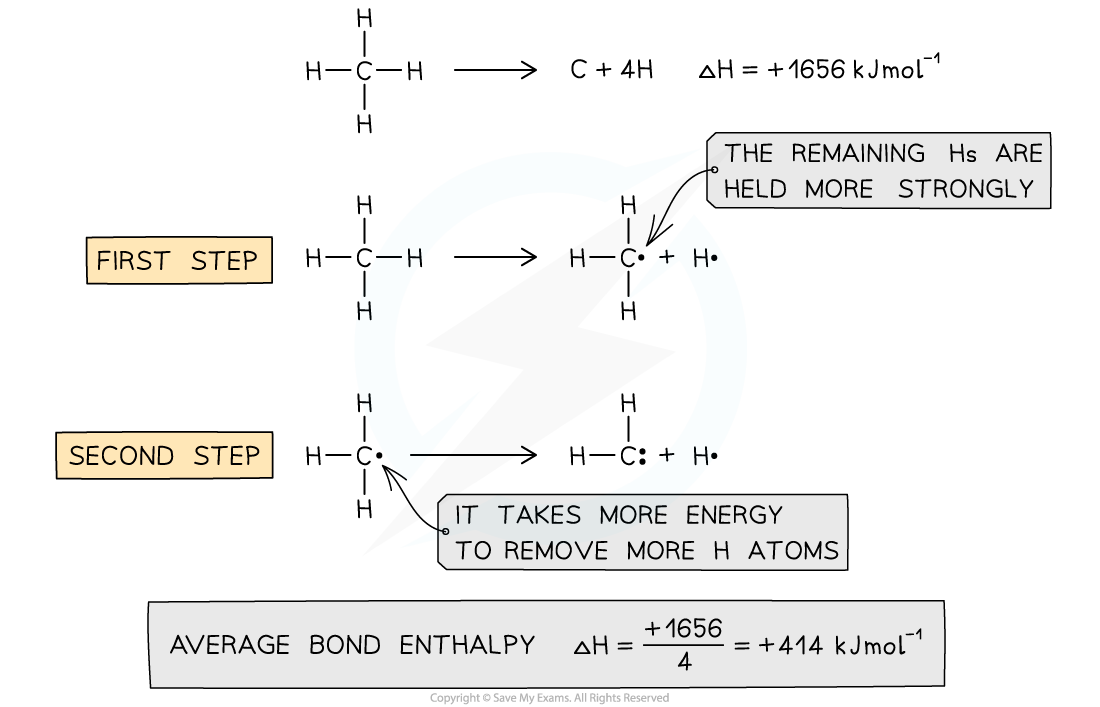# IB DP Chemistry: SL复习笔记5.3.1 Bond Enthalpy

### Bonds & Energy

• When bonds are broken or made enthalpy changes take place
• A chemical bond is a force of attraction between two atoms
• Breaking the bond requires the input of energy it is therefore an endothermic process
• The energy change required to break the bond depends on the atoms that form the bond
• The energy required to break a particular bond is called the bond dissociation enthalpy
• This is usually just shortened to bond enthalpy or bond energy
• Bond formation is the opposite of bond breaking and so energy is released when bonds are formed
• It is therefore an exothermic processTo break bonds energy is required from the surroundings and to make new bonds energy is released from the reaction to the surroundings

• The amount of energy released when a particular bond is formed has the same magnitude as the energy taken in when the bond is broken but has the opposite sign

Overall enthalpy changes

• If more energy is released when new bonds are formed than energy is required to break bonds, the reaction is exothermic
• The products are more stable than the reactants
• If more energy is required to break bonds than energy is released when new bonds are formed, the reaction is endothermic
• The products are less stable than the reactants
• The relationship between bond breaking and bond making can be shown graphically like this:Bond enthalpy profiles

### Bond Enthalpy

#### Average bond energy

• Bond energies are affected by other atoms in the molecule (the environment)
• Therefore, an average of a number of the same type of bond but in different environments is calculated
• This bond energy is known as the average bond energy and is defined as

'The energy needed to break one mole of bonds in a gaseous molecule averaged over similar compounds'Average bond enthalpy of C-H in methane

• The average bond enthalpy of C-H is found by taking the bond dissociation enthalpy for the whole molecule and dividing it by the number of C-H bonds
• The first C-H bond is easier to break than the second as the remaining hydrogens are pulled more closely to the carbon
• However, since it is impossible to measure the energy of each C-H bond an average is taken
• This value is also compared with a range of similar compounds to obtain an accepted value for the average bond enthalpy

#### Exam Tip

A lot of students mix up endothermic / exothermic and bond breaking / bond making.

An easy way to remember is that ENDOTHERMIC leads to the poetic phrase the 'end o' the bond'